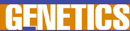# Chi-Square Test - Research Article from World of Genetics

This encyclopedia article consists of approximately 5 pages of information about Chi-Square Test.
 This section contains 1,353 words (approx. 5 pages at 300 words per page) View a FREE sampleThe chi-square test (K2) is the most commonly used method for comparing frequencies or proportions. It is a statistical test used to compare observed data with data that would be expected according to a given hypothesis. A statistical test to determine if the observed numbers deviate from those expected under a particular hypothesis. The chi-square test is also referred to as a test of a measure of fit or "goodness of fit" between data. Typically, the hypothesis tested is whether or not two samples are different enough in a particular characteristic. Chi-square tests evaluate data belonging to a nominal scale, that is, a scale used when the values fit into categories that describe a quality of the person, including gender or morphological characteristics. Chi-square analysis belongs to the family of univariate analysis, i.e., those tests able to evaluate the possible effect of one variable (often...

 This section contains 1,353 words (approx. 5 pages at 300 words per page) View a FREE sample